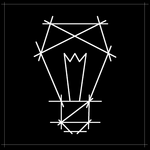Community Profile# Ashutosh Rout

Last seen: 1 year ago Active since 2018

#### Statistics

All
•••••#### Content Feed

View by

Submitted

Convolution of periodic sequence using DFT & IDFT.
DFT:Discrete Fourier Transform It computes N equally spaced frequency samples of the DTFT. IDFT:Inverse Discrete Fourier Transfo...Submitted

Power Spectral Density of a sequence x(n)using N-point DFT
The power spectral density (PSD) of the signal describes the power present in the signal as a function of frequency, per unit fr...Solved

Weighted average
Given two lists of numbers, determine the weighted average. Example [1 2 3] and [10 15 20] should result in 33.333...

4 years ago

Solved

Is my wife right?
Regardless of input, output the string 'yes'.

4 years ago

Solved

Given a and b, return the sum a+b in c.

4 years ago

Solved

Times 2 - START HERE
Try out this test problem first. Given the variable x as your input, multiply it by two and put the result in y. Examples:...

4 years ago

Solved

Find the sum of all the numbers of the input vector
Find the sum of all the numbers of the input vector x. Examples: Input x = [1 2 3 5] Output y is 11 Input x ...

4 years ago

Solved

Make the vector [1 2 3 4 5 6 7 8 9 10]
In MATLAB, you create a vector by enclosing the elements in square brackets like so: x = [1 2 3 4] Commas are optional, s...

4 years ago

Submitted

Circular-convolution with using cconv(x,y,n)
Circular convolution is the fundamental operation to compute discrete time signals.Submitted

Circular-convolution using fft(x) and ifft(X)
Circular convolution using properties of Discrete Fourier Transform.Submitted

Circular-convolution without using cconv(x,y,n)
It is used to convolve two Discrete Fourier transform sequences.It is faster for long sequences than linear convolution.Submitted

Auto-correlation of a sequence x without using xcorr(x)
It is the correlation of a signal with a delayed copy of itself as a function of delay.Submitted

Cross-correlation sequences x and y without using xcorr(x,y)
Cross-correlation measures the similarity between x and shifted (lagged) copies of y as a function of the lag.